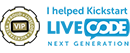## Parse to number error

Moderators: LCMark, LCfraser

pink
Posts: 252
Joined: Wed Mar 12, 2014 6:18 pm

### Parse to number error

I am trying to create a LCB handler that converts a number from base 16 to base 10, however, it is erroring out at the line where I parse to a number...

error: Parsing error: syntax error
parse tChar as Number into tNum
^
5:36 PM: Error: failed to compile module

below is my actual code:

Code: Select all

``````public handler hexToDec(in pText as String)
variable tChar as optional String
variable tNum as optional Number
variable tSum as Number
variable tCount as Number
variable tHex as Number

put the number of chars in pText into tCount
repeat for each char tChar in pText
subtract 1 from tCount
if tChar is "A" then
put "10" into tChar
else if tChar is "B" then
put "11" into tChar
else if tChar is "C" then
put "12" into tChar
else if tChar is "D" then
put "13" into tChar
else if tChar is "E" then
put "14" into tChar
else if tChar is "F" then
put "15" into tChar
end if

parse tChar as Number into tNum
add (tNum * (16^tCount)) to tSum
end repeat
return tSum
end handler``````
Greg (pink) Miller

I'm Mad, Pink and Dangerous to Know

pink
Posts: 252
Joined: Wed Mar 12, 2014 6:18 pm

### Re: Parse to number error

I've even tried using the examples from the ParseStringAsNumber, both of them give me the same error when testing

Code: Select all

``````public handler practiceMe()
variable tString as String
put "5.6" into tString
parse tString as Number -- the result is 5.6
end handler

public handler practiceMe2()
variable tResult as optional String
variable tNum as optional Number
parse "aaaa" as Number into tNum

if tNum is nothing then
put "unable to parse string" into tResult
end if
end handler``````
Greg (pink) Miller

I'm Mad, Pink and Dangerous to Know

bn
VIP Livecode Opensource BackerPosts: 3325
Joined: Sun Jan 07, 2007 9:12 pm
Location: Bochum, Germany

### Re: Parse to number error

Hi Greg,

the problem here is that "as Number" has to be lower case "as number". Uppercase is for casting types in variable declarations.
Note "log" which is very helpful for debugging. You can log anything if you put it into square brackets [] like
log [tVariable1, tVariable2], for strings you can omit the square brackets.

I modified your scripts and they compile and work if called. I tested in LC 9.0.1 rc1.

Code: Select all

``````private handler practiceMe()
variable tString as String
variable tNum as Number
put "5.6" into tString
parse tString as number into tNum -- the result is 5.6
log [tNum]
end handler

private handler practiceMe2()
variable tResult as optional String
variable tNum as optional Number
parse "aaaa" as number into tNum

if tNum is nothing then
put "unable to parse string" into tResult
log tResult
end if
end handler``````
Kind regards
Bernd

[-hh]
VIP Livecode Opensource BackerPosts: 2192
Joined: Thu Feb 28, 2013 11:52 pm
Location: Göttingen, DE

### Re: Parse to number error

You could also try the following.

Code: Select all

``````handler hexToDec(in pText as String) returns Number
variable tChar as String
variable hexChars as String
variable tNum as Number
variable tSum as Number
variable tCount as Number
put 0 into tSum
put the number of chars in pText into tCount
put "0123456789ABCDEF" into hexChars
repeat for each char tChar in pText
subtract 1 from tCount
put -1+the offset of tChar in hexChars into tNum
add (tNum * (16^tCount)) to tSum
end repeat
return tSum
end handler``````
Variant:

Code: Select all

``````handler hexToDec(in pText as String) returns Number
variable tChar as String
variable hexList as List
variable tNum as Number
variable tSum as Number
variable tCount as Number
put 0 into tSum
put ["0","1","2","3","4","5","6","7","8","9","A","B","C","D","E","F"] into hexList
put the number of chars in pText into tCount
repeat for each char tChar in pText
subtract 1 from tCount
put -1+the index of tChar in hexList into tNum
add (tNum * (16^tCount)) to tSum
end repeat
return tSum
end handler``````
shiftLock happens

pink
Posts: 252
Joined: Wed Mar 12, 2014 6:18 pm

### Re: Parse to number error

Thank you both... of course I finally found the "BaseConvert" command in the dictionary.

Unfortunately I copied these examples right out of the dictionary, will need to get that fixed
Greg (pink) Miller

I'm Mad, Pink and Dangerous to Know

[-hh]
VIP Livecode Opensource BackerPosts: 2192
Joined: Thu Feb 28, 2013 11:52 pm
Location: Göttingen, DE

### Re: Parse to number error

The built-in "baseConvert" returns a string, not a number (and is limited to base 32).
So, nevertheless, you need the "parse as number" (or for bases > 32 the above offset-technique).
shiftLock happens